# EP2515142A2 - Maximum likelihood angle estimation of wideband signals using phased array antennas - Google Patents

Maximum likelihood angle estimation of wideband signals using phased array antennas Download PDF

## Info

Publication number
EP2515142A2
EP2515142A2 EP12155386A EP12155386A EP2515142A2 EP 2515142 A2 EP2515142 A2 EP 2515142A2 EP 12155386 A EP12155386 A EP 12155386A EP 12155386 A EP12155386 A EP 12155386A EP 2515142 A2 EP2515142 A2 EP 2515142A2
Authority
EP
European Patent Office
Prior art keywords
angle
stabilization
spatial frequency
frequency data
steering
Prior art date
Legal status (The legal status is an assumption and is not a legal conclusion. Google has not performed a legal analysis and makes no representation as to the accuracy of the status listed.)
Withdrawn
Application number
EP12155386A
Other languages
German (de)
French (fr)
Other versions
EP2515142A3 (en
Inventor
Charles T. Hansen
Current Assignee (The listed assignees may be inaccurate. Google has not performed a legal analysis and makes no representation or warranty as to the accuracy of the list.)
Raytheon Co
Original Assignee
Raytheon Co
Priority date (The priority date is an assumption and is not a legal conclusion. Google has not performed a legal analysis and makes no representation as to the accuracy of the date listed.)
Filing date
Publication date
Priority to US13/092,073 priority Critical patent/US8451173B2/en
Application filed by Raytheon Co filed Critical Raytheon Co
Publication of EP2515142A2 publication Critical patent/EP2515142A2/en
Publication of EP2515142A3 publication Critical patent/EP2515142A3/en
Withdrawn legal-status Critical Current

• 238000007476 Maximum Likelihood Methods 0.000 title description 7
• 239000011159 matrix materials Substances 0.000 claims abstract description 16
• 230000000087 stabilizing Effects 0.000 claims abstract description 10
• 239000003381 stabilizers Substances 0.000 claims description 9
• 238000010606 normalization Methods 0.000 claims description 2
• 238000000034 methods Methods 0.000 description 14
• 238000010586 diagrams Methods 0.000 description 9
• 230000000875 corresponding Effects 0.000 description 3
• 230000001131 transforming Effects 0.000 description 3
• 238000004422 calculation algorithm Methods 0.000 description 2
• 230000014509 gene expression Effects 0.000 description 2
• 239000000203 mixtures Substances 0.000 description 2
• 238000002940 Newton-Raphson method Methods 0.000 description 1
• 239000003570 air Substances 0.000 description 1
• 230000005540 biological transmission Effects 0.000 description 1
• 238000004364 calculation methods Methods 0.000 description 1
• 239000000969 carriers Substances 0.000 description 1
• 238000009795 derivation Methods 0.000 description 1
• 238000005315 distribution function Methods 0.000 description 1
• 230000004048 modification Effects 0.000 description 1
• 238000006011 modification reactions Methods 0.000 description 1
• 238000001228 spectrum Methods 0.000 description 1

## Images

•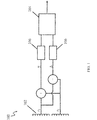•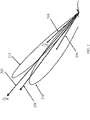•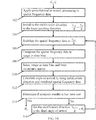•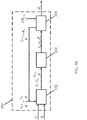••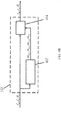•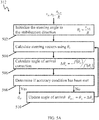•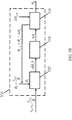## Classifications

• HELECTRICITY
• H01BASIC ELECTRIC ELEMENTS
• H01Q3/00Arrangements for changing or varying the orientation or the shape of the directional pattern of the waves radiated from an antenna or antenna system
• GPHYSICS
• G01MEASURING; TESTING
• G01S13/00Systems using the reflection or reradiation of radio waves, e.g. radar systems; Analogous systems using reflection or reradiation of waves whose nature or wavelength is irrelevant or unspecified
• G01S13/02Systems using reflection of radio waves, e.g. primary radar systems; Analogous systems
• GPHYSICS
• G01MEASURING; TESTING
• G01S13/00Systems using the reflection or reradiation of radio waves, e.g. radar systems; Analogous systems using reflection or reradiation of waves whose nature or wavelength is irrelevant or unspecified
• G01S13/02Systems using reflection of radio waves, e.g. primary radar systems; Analogous systems
• G01S13/0209Systems with very large relative bandwidth, i.e. larger than 10 %, e.g. baseband, pulse, carrier-free, ultrawideband
• GPHYSICS
• G01MEASURING; TESTING
• G01S13/00Systems using the reflection or reradiation of radio waves, e.g. radar systems; Analogous systems using reflection or reradiation of waves whose nature or wavelength is irrelevant or unspecified
• G01S13/02Systems using reflection of radio waves, e.g. primary radar systems; Analogous systems
• G01S13/06Systems determining position data of a target
• G01S13/42Simultaneous measurement of distance and other co-ordinates
• GPHYSICS
• G01MEASURING; TESTING
• G01S13/00Systems using the reflection or reradiation of radio waves, e.g. radar systems; Analogous systems using reflection or reradiation of waves whose nature or wavelength is irrelevant or unspecified
• G01S13/02Systems using reflection of radio waves, e.g. primary radar systems; Analogous systems
• G01S13/06Systems determining position data of a target
• G01S13/42Simultaneous measurement of distance and other co-ordinates
• G01S13/44Monopulse radar, i.e. simultaneous lobing
• G01S13/4454Monopulse radar, i.e. simultaneous lobing phase comparisons monopulse, i.e. comparing the echo signals received by an interferometric antenna arrangement
• GPHYSICS
• G01MEASURING; TESTING
• G01S3/00Direction-finders for determining the direction from which infrasonic, sonic, ultrasonic, or electromagnetic waves, or particle emission, not having a directional significance, are being received
• G01S3/02Direction-finders for determining the direction from which infrasonic, sonic, ultrasonic, or electromagnetic waves, or particle emission, not having a directional significance, are being received using radio waves
• G01S3/14Systems for determining direction or deviation from predetermined direction
• G01S3/28Systems for determining direction or deviation from predetermined direction using amplitude comparison of signals derived simultaneously from receiving antennas or antenna systems having differently-oriented directivity characteristics
• G01S3/32Systems for determining direction or deviation from predetermined direction using amplitude comparison of signals derived simultaneously from receiving antennas or antenna systems having differently-oriented directivity characteristics derived from different combinations of signals from separate antennas, e.g. comparing sum with difference
• GPHYSICS
• G01MEASURING; TESTING
• G01S3/00Direction-finders for determining the direction from which infrasonic, sonic, ultrasonic, or electromagnetic waves, or particle emission, not having a directional significance, are being received
• G01S3/02Direction-finders for determining the direction from which infrasonic, sonic, ultrasonic, or electromagnetic waves, or particle emission, not having a directional significance, are being received using radio waves
• G01S3/14Systems for determining direction or deviation from predetermined direction
• G01S3/46Systems for determining direction or deviation from predetermined direction using antennas spaced apart and measuring phase or time difference between signals therefrom, i.e. path-difference systems
• GPHYSICS
• G01MEASURING; TESTING
• G01S7/00Details of systems according to groups G01S13/00, G01S15/00, G01S17/00
• G01S7/02Details of systems according to groups G01S13/00, G01S15/00, G01S17/00 of systems according to group G01S13/00
• G01S7/28Details of pulse systems
• G01S7/295Means for transforming co-ordinates or for evaluating data, e.g. using computers

## Abstract

A method for estimating a target direction of a wideband signal received on an electronically steered array includes: applying convolutional or stretch processing to spatial frequency data of the wideband signal; initializing a stabilization direction to a beam pointing direction; stabilizing the spatial frequency data to the stabilization direction; compressing the spatial frequency data to a plurality of frequency range or time bins; selecting range or time bins and forming a covariance matrix; calculating an estimated target direction using the covariance matrix; determining if a stabilization direction accuracy condition is met; recalculating the stabilization direction based on the estimated target direction if the stabilization direction accuracy condition is not met; and iteratively repeating until the stabilization direction accuracy condition is met.

## Description

STATEMENT REGARDING FEDERALLY SPONSORED RESEARCH OR DEVELOPMENT
• This invention disclosure is related to Government contract FA8650-08-D-1446 awarded by the Department of the Air Force. The Government has certain rights in this invention.
• BACKGROUND
• Maximum likelihood angle estimation is a technique which may be used in the context of radar for determining an angle of arrival of a signal in a radar or other radio frequency electromagnetic sensor.
• Generally, the algorithm that performs the angle estimation using maximum likelihood estimation relies on the assumption that the estimated target angle does not substantially vary as a function of the frequencies that make up the signal. However, this assumption does not hold true for wideband or spread spectrum waveforms or signals when they are measured by an electronically scanned phased array antenna.
• SUMMARY
• Embodiments of the present invention are directed to systems and methods which use maximum likelihood analysis to estimate a target angle, the systems and methods being substantially frequency invariant even when used with phase steered antennas and wideband waveforms.
• According to one embodiment of the present invention, a method for estimating a target direction of a wideband signal received on an electronically steered array includes: applying convolutional or stretch processing to spatial frequency data of the wideband signal; initializing a stabilization direction to a beam pointing direction; stabilizing the spatial frequency data to the stabilization direction; compressing the spatial frequency data to a plurality of frequency range or time bins; selecting range or time bins and forming a covariance matrix; calculating an estimated target direction using the covariance matrix; determining if a stabilization direction accuracy condition is met; recalculating the stabilization direction based on the estimated target direction if the stabilization direction accuracy condition is not met; and iteratively repeating stabilizing the spatial frequency data to the stabilization direction, compressing the spatial frequency data to a plurality of frequency range or time bins, calculating an estimated target direction using the covariance matrix, and recalculating the stabilization direction based on the estimated target direction until the stabilization direction accuracy condition is met.
• According to another embodiment of the present invention, a system for stabilizing wideband spatial frequency data of a wideband signal for estimating a target direction on an electronically steered antenna array includes: a beam stabilizer configured to stabilize the spatial frequency data in a stabilization direction; a steering angle calculator configured to estimate the target direction using the stabilized spatial frequency data; a stabilization angle accuracy threshold calculator for determining whether a stabilization direction accuracy condition is met; and a stabilization angle updater for updating the stabilization direction to the estimated target direction, wherein the steering angle calculator is configured to initialize the stabilization direction to a beam pointing direction and to iteratively update the stabilization angle and to recalculate the estimated target direction until the stabilization accuracy condition is met.
• BRIEF DESCRIPTION OF THE DRAWINGS
• The accompanying drawings, together with the specification, illustrate exemplary embodiments of the present invention, and, together with the description, serve to explain the principles of the present invention.
• FIG. 1 is a block diagram of a radar system according to one embodiment of the present invention.
• FIG. 2 is a diagram illustrating various directions or angles associated with the system, including a nominal beam pointing direction, a target direction, and a stabilization direction, overlaid on target angles calculated using an unstabilized beam.
• FIG. 3A is a flowchart illustrating a method of determining an angle to a target from unstabilized spatial frequency data according to one embodiment of the present invention.
• FIG. 3B is a block diagram illustrating a signal processor for determining a target angle from unstabilized spatial frequency data according to one embodiment of the present invention.
• FIG. 4A is a flowchart illustrating a method of stabilizing spatial frequency data according to one embodiment of the present invention.
• FIG. 4B is a block diagram illustrating a signal stabilizer according to one embodiment of the present invention.
• FIG. 5A is a flowchart illustrating a method of calculating a steering angle according to one embodiment of the present invention.
• FIG. 5B is a block diagram illustrating a steering angle calculator according to one embodiment of the present invention.
DETAILED DESCRIPTION
• According to one embodiment of the present invention, maximum likelihood angle estimation may be used in a radar system 100 such as one shown in block diagram form in FIG. 1. An antenna 102 receives a signal which is divided into two components (s 1, and s 2) split into sum and difference channels (Σ and Δ) and supplied to analog-to-digital converters 106. A signal processor 104 may be used to analyze the received signals. The system 100 may also include filters, oscillators, and other analog and digital signal processing components as are well known in the art.
• Angle estimation of radar returns is often performed with an antenna having two channels: a sum channel and a difference channel. The angle of arrival of a received signal (or, equivalently, due to antenna reciprocity theorem, the transmission angle of an outgoing signal) is computed using the sum and difference channels, but the relationship between the angle of arrival and the relative amplitude of the received signal depends on the frequency of the signal when the antenna is steered by adjusting the phase between the elements. As a result, these angle estimation techniques are generally used with narrowband signals rather than wideband signals because there is not a well defined mapping between angle and relative amplitude of the signal for wideband signals. That is, the wideband signals have a range of frequencies, making it difficult to calculate a single angle of arrival for all of the frequencies. For example, as shown in FIG. 2, different target angles 210 and 212 may be calculated based at opposing ends of the wideband signal. Therefore, a substantially frequency independent method of determining the angle of arrival would be useful.
• One such system and method is described in "Beam Stabilization for Wideband Phase Comparison Monopulse Angle Estimation with Electronically Steered Antennas," U.S. Patent Application No. 12/925,668 filed on October 26, 2010 , the entire disclosure of which is incorporated herein by reference.
• Aspects of a maximum likelihood method and system according to embodiments of the present invention are directed to substantially reducing or eliminating deterministic high order angle estimation error caused by beam stabilization in frequency and providing a way to combine multiple observations (e.g., in the case of a radar signal, multiple range or time bins) into a single angle estimate, which can provide an effective reduction of random errors by an improvement in signal to noise ratio.
• Stabilization of the beam in frequency space so that frequency elements of the beam point in a consistent direction is used in order to apply the angle estimation technique to wideband signals. Beam stabilization is discussed in more depth in the above referenced patent application. To summarize, the matrix elements for beam stabilization as a function of frequency are given by: $m ΣΔ = - s Δ ʹ ⁢ D s Σ ʹ ∂ s Δ ʹ - s Δ ʹ ∂ s Σ ʹ$ $m ΔΔ = D - m ΔΣ ∂ s Σ ʹ ∂ s Δ ʹ$ $m ΣΔ = - ∂ s Σ ʹ ⁢ S s Σ ʹ ∂ s Δ ʹ - s Δ ʹ ∂ s Σ ʹ$ $m ΣΣ = S - m ΣΔ ⁢ s Δ ʹ s Σ ʹ$
• Assuming we are only solving for on angle of arrival, in the above expressions: $S = s ∑ x ʹ x o ⁢ k o$
and $D = ∂ s ∑ x ʹ x o ⁢ k o$
where we have defined: $s ∑ ʹ = s ∑ x ʹ x o ⁢ k = ∑ n w ∑ xn exp i x o R ⁢ d xn ⁢ k - k o$ $∂ s ∑ ʹ = ∂ s ∑ x ʹ ∂ x x o ⁢ k = ∑ n w ∑ xn d xn ⁢ k R ⁢ exp i x o R ⁢ d xn ⁢ k - k o$
and $Δ Δ ʹ = Δ Δ ⁢ x ʹ x o ⁢ k = ∑ n w Δ ⁢ xn exp i x o R ⁢ d xn ⁢ k - k o$ $∂ Δ Δ ʹ = ∂ Δ Δ ⁢ x ʹ ∂ x x o ⁢ k = ∑ n w Δ ⁢ xn d xn ⁢ k R ⁢ exp i x o R ⁢ d xn ⁢ k - k o$
where when two signals (s 1, and s 2) are received on corresponding halves of an antenna, s' is a sum channel and s' Δ is a difference channel (e.g., $s ∑ ′ = s 1 + s 2$ and $s Δ ′ = s 1 - s 2$ ), $k = ω c$ is the spatial frequency, dxn are the element locations, $x o R$ is the desired pointing direction cosine, $k o = ω o c$ is the nominal spatial frequency corresponding to the radio frequency carrier, and W ∑,Δxn are the stabilized amplitudes of the radiating elements that form the phased array antenna. W ∑,Δxn can be found from: $w Σ , Δ ⁢ xn = ∑ n w Σ , Δ ⁢ xn ʹ exp i ⁢ x o R - x p R ⁢ d xn ⁢ k o$
where W' ∑,Δxn are the unsteered amplitudes of the radiating elements that form the phased array antenna and $x p R$ is the direction cosine defined by the steering phases commanded by the antenna steering hardware. Note that the beam is not necessarily stabilized to the pointing direction commanded by the hardware. Therefore, in general, xo xp.
• A derivation of the above equations appears in "Beam Stabilization for Wideband Phase Comparison Monopulse Angle Estimation with Electronically Steered Antennas," which was incorporated by reference, above.
• Note that there are a few different relevant angles (or "directions") involved in this system, as illustrated in FIG. 2:
• The "antenna pointing direction" 202 $x p R$ refers to the direction in which the antenna is electronically steered by altering the phases of the signals applied to the antenna.
• The "stabilization direction" 204 $x o R$ refers to the direction in which the beam is stabilized to be substantially frequency independent through the computation of and application of the above-described stabilization coefficients (e.g., m Δ∑ , m ΔΔ, m Δ∑ , and m ∑∑) to the unstabilized data. The stabilization direction is not restricted to the antenna pointing direction and in one embodiment is adjusted to optimize angle estimation.
• The "steering direction" 206 (θ n ) refers to the direction which is used to calculate the likelihood function for the purpose of angle estimation.
• The "target direction" 208 refers to the true direction to the target, which the system and method attempt to estimate.
• The maximum likelihood angle estimation technique applies probability theory to determine the most probable angle of arrival of the signal. In particular, the most probable angle of arrival of the signal occurs at the maximum of the following likelihood function (which is related to the logarithm of the probability distribution function): $J θ = ∑ n | s † θ ⁢ Q - 1 ⁢ y n | 2 s † θ ⁢ Q - 1 ⁢ s θ$ where yn is the set of stabilized data vectors from the antennas, which includes s and s Δ, s(θ) is the steering vector corresponding to an angle θ ("steering direction" as defined above), and Q is the covariance matrix of any undesired signals present, such as noise, interference, or clutter.
• The steering vector s(θ) can be written: $s θ = ∑ n w Σ ⁢ xn ʹ exp i ⁢ θ - x o R ⁢ d xn ⁢ k o ∑ n w Δ ⁢ xn ʹ exp i ⁢ θ - x o R ⁢ d xn ⁢ k o$
where, in this case, θ is the likelihood steering direction cosine. Recall that $x o R$ is the direction cosine to which the beam is stabilized.
• The likelihood function can be rewritten: $J θ = ∑ n s † θ ⁢ Q - 1 y n y n † ⁢ Q - 1 ⁢ s θ s † θ ⁢ Q - 1 ⁢ s θ$
where we have used the fact that Q is Hermetian. Because only yn depends on n, the summation can be brought inside the product: $J θ = s † θ ⁢ Q - 1 ∑ n ⁢ y n y n † ⁢ Q - 1 ⁢ s θ s † θ ⁢ Q - 1 ⁢ s θ$
• Therefore, the covariance matrix of the desired signals is: $∑ n y n y n † ≡ C y$
• Therefore, the likelihood function when multiple observations or range or time bins are present depends only on the covariance matrix of the data in question.
• The maximum of the likelihood function can be found by finding the zero of its derivative. This may be found using a Newton-Raphson technique. The derivative of the likelihood function is: $Jʹ θ = ∑ n 2 ⁢ s † θ ⁢ Q - 1 ⁢ s θ ⁢ s † θ ⁢ Q - 1 ⁢ y n ⁢ y n † ⁢ Q - 1 ⁢ ∂ s θ ∂ θ - 2 ⁢ s † θ ⁢ Q - 1 ⁢ y n ⁢ y n † ⁢ Q - 1 ⁢ y n ⁢ y n † ⁢ Q - 1 ⁢ s θ ⁢ s † θ ⁢ Q - 1 ⁢ ∂ s θ ∂ θ s † θ ⁢ Q - 1 ⁢ s θ 2$
which is zero when the numerator is zero. Therefore, we would like to find the zero of: $f θ = ∑ n ⁢ s † θ ⁢ Q - 1 ⁢ s θ ⁢ s † θ ⁢ Q - 1 y n y n † ⁢ Q - 1 ⁢ ∂ s θ ∂ θ - s † θ ⁢ Q - 1 y n y n † ⁢ Q - 1 ⁢ s θ ⁢ s † θ ⁢ Q - 1 ⁢ ∂ s θ ∂ θ$
• Near its zero, this function can be approximated to first order by $f θ ≈ f θ n + fʹ θ n ⁢ θ - θ n$
where: $fʹ θ = ∂ s † θ ∂ θ ⁢ Q - 1 ⁢ s θ ⁢ s † θ ⁢ Q - 1 C y Q - 1 ⁢ ∂ s θ ∂ θ + s † θ ⁢ Q - 1 ⁢ ∂ s θ ∂ θ ⁢ s † θ ⁢ Q - 1 ⁢ C y ⁢ Q - 1 ⁢ ∂ s θ ∂ θ + s † θ ⁢ Q - 1 ⁢ s θ ⁢ ∂ s † θ ∂ θ ⁢ Q - 1 ⁢ C y ⁢ Q - 1 ⁢ ∂ s θ ∂ θ + s † θ ⁢ Q - 1 ⁢ s θ ⁢ s † θ ⁢ Q - 1 ⁢ C y ⁢ Q - 1 ⁢ ∂ 2 ⁢ s θ ∂ θ 2 - ∂ s † θ ∂ θ ⁢ Q - 1 ⁢ C y ⁢ Q - 1 ⁢ s θ ⁢ s † θ ⁢ Q - 1 ⁢ ∂ s θ ∂ θ - s † θ ⁢ Q - 1 ⁢ C y ⁢ Q - 1 ⁢ ∂ s θ ∂ θ ⁢ s † θ ⁢ Q - 1 ⁢ ∂ s θ ∂ θ - s † θ ⁢ Q - 1 ⁢ C y ⁢ Q - 1 ⁢ s θ ⁢ ∂ s † θ ∂ θ ⁢ Q - 1 ⁢ ∂ s θ ∂ θ - s † θ ⁢ Q - 1 ⁢ C y ⁢ Q - 1 ⁢ s θ ⁢ s † θ ⁢ Q - 1 ⁢ ∂ s θ ∂ θ 2$
• Therefore, the Newton-Raphson iterative procedure is: $θ n + 1 = θ n - f θ n fʹ θ n$
• That is, the Newton-Raphson iterative procedure is used to produce successive values of θ n until the calculated angle of arrival $Δ ⁢ θ n = - f θ n fʹ θ n$ correction (or improvement) is less than a threshold Δθ n,th, at which point an estimated target angle θ N is output.
• However, the results calculated from this procedure will retain some error due to the beam stabilization transformation. To reduce or eliminate this error, the direction of beam stabilization can be adjusted to the newly determined steering angle and the Newton-Raphson iterative procedure can be repeated using the re-stabilized data to calculate a steering angle that is a more accurate estimate of the actual angle of arrival of the signal. This process of iteratively adjusting the stabilization direction can be repeated until a stabilization direction accuracy condition has been met (e.g., the difference between successively calculated estimated target angles θ N is less than Δθ N,th ), at which point the final estimated target angle is output.
• According to one embodiment of the present invention, a method for implementing the above technique can be performed as follows and as illustrated in FIG. 3A. A system according to one embodiment of the present invention that may be configured to implement the algorithm of FIG. 3A is shown in FIGS. 4B, 5B, and 5B.
• FIG. 3A is a flowchart illustrating one method of determining an angle to a target from unstabilized spatial frequency data which is formed after initial range processing of the raw data received from an antenna. FIG. 3B is a block diagram illustrating a portion of a signal processor 104 for determining the target angle from the spatial frequency data. The signal received from the antenna undergoes transformation into spatial frequency data (e.g., through the first stage of convolutional or stretch processing) (302) at a beam stabilizer 332.
• Initially, the direction in which the beam is stabilized $x o R$ is set to (or initialized to) the nominal pointing direction of the antenna array $x p R ,$ which is the pointing direction associated with the center frequency of the waveform (304). In other words, $x o , 0 R = x p R ,$ where $x o , n R$ refers to the beam stabilization direction during the nth iteration of the loop. However, the stabilization direction is generally different from the antenna pointing direction.
• The unstabilized spatial frequency data s' Δ and s' is stabilized in the beam stabilization direction $x o , n R$ (306) to produce stabilized spatial frequency data s Δ and s , after which it is compressed into range or time bins (308) by the beam stabilizer 332. Range or time bins are selected and a covariance matrix is also computed (310) by the beam stabilizer 332. The stabilized spatial frequency data s Δ and s is then supplied with the beam stabilization direction to the steering angle calculator 334.
• The steering angle calculator 334 calculates an angle of arrival θ N using the stabilization direction and the stabilized spatial frequency data (312). As noted above, this calculation results in deterministic error due to the beam stabilization transformation. In order to reduce or eliminate this error, a stabilization direction threshold accuracy calculator 336 compares the newly calculated estimated angle of arrival θ N with the previous estimated angle of arrival (e.g., θ N-1 ) to determine if a stabilization direction accuracy condition has been met (e.g., the difference between two successive estimated angles of arrival is less than a threshold value Δθ N,th ) (314). If the stabilization direction accuracy condition is not satisfied, then the stabilization direction is set to the newly calculated estimated angle of arrival (316) and the process is repeated, beginning with the restabilization of the beam in the new stabilization direction (312) by supplying the new stabilization direction to the beam stabilizer 332. When the stabilization direction accuracy condition is satisfied, the newly computed estimated angle of arrival θ N is output as the angle to the target. When working with multiple targets, a separate data covariance matrix is determined for each target. For example, if two targets are separated in range, two different groups of range or time bins would be used to form two different covariance matrices.
• FIG. 4A is a flowchart illustrating, in additional detail, one method of stabilizing the spatial frequency data to the beam steering direction. FIG. 4B is a block diagram illustrating a beam stabilizer 332 according to one embodiment of the present invention. The spatial frequencies (e.g., s' Δ and s' ) are calculated from the spatial frequency data (402) using processing parameters of the signal waveforms (e.g., the characteristics of the transmitted pulse) and the antenna direction. Using antenna parameters (e.g., the number and spacing of antenna elements), antenna pattern values (e.g., element amplitudes or weights of the antenna elements) and derivatives of the spatial frequencies are also calculated (404). Normalization constants are used to solve for stabilization coefficients (e.g., m Δ∑, m ΔΔ , m ∑Δ , and m ∑∑, as described above) (406) by a stabilization coefficient calculator 432. The stabilization coefficients (which may be expressed as a combination matrix) are applied to the spatial frequency data (e.g., s' Δ and s' ) by a combiner (or matrix multiplier) 434 to obtain the stabilized spatial frequency data stabilized in the stabilization direction (e.g., s Δ = m ΔΔ s' Δ + m Δ∑ s' and s = m ∑Δ s' Δ + m ∑∑ s' ) (408).
• FIG. 5A is a flowchart illustrating a method of calculating a steering angle according to one embodiment of the present invention. FIG. 5B is a block diagram illustrating a steering angle calculator 334 according to one embodiment of the present invention which uses the Newton-Raphson method described above to estimate the angle of arrival.
• The steering angle calculator 334 includes a steering vector calculator 532 which initializes the steering vectors θ n to the stabilization direction $x o , n R$ (e,g., $θ 0 = x o , n R )$ (502) and calculates steering vectors using the stabilized spatial frequency data s Δ and s Σ (504). An angle correction calculator 534 then uses the steering vectors s n ) to calculate an angle of arrival correction Δθ n (506), where, as derived above: $Δ ⁢ θ n = f θ n fʹ θ n$
• An angle of arrival accuracy calculator 536 compares the angle of arrival correction Δθ n with an angle of arrival accuracy condition Δθ n,th (508). If the angle of arrival accuracy condition is not satisfied (e.g., Δθ n > Δθ n,th ), a new estimate of the angle of arrival θ n+1 is computed by applying the Newton-Raphson iterative procedure described above using the expression: $θ n + 1 = θ n + Δ ⁢ θ n$
and the improved target angle estimate θ n+1 is supplied to the steering vector calculator 532 in order to generate new steering vectors (e.g., s n+1 )) (510). This process is repeated until the angle of arrival accuracy condition Δθ n,th is met and the estimate of the target angle θ N is output by the steering angle calculator 334.
• While the present invention has been described in reference to certain exemplary embodiments, it is to be understood to those skilled in the art that the invention is not limited to the disclosed embodiment, but, on the contrary, is intended to cover various modifications included within the spirit and scope of the appended claims and equivalents thereof.
• For example, individual hardware components (such as those shown in the embodiments depicted in FIGS. 1, 4B, 5B, and 5B) may be implemented as specialized discrete chips (such as digital signal processors, analog or digital filters, and field programmable gate arrays), general purpose processors programmed to perform the functions of the shown components, or combinations thereof.

## Claims (9)

1. A method for estimating a target direction of a wideband signal received on an electronically steered array, the method comprising:
applying convolutional or stretch processing to spatial frequency data of the wideband signal;
initializing a stabilization direction to a beam pointing direction;
stabilizing the spatial frequency data to the stabilization direction;
compressing the spatial frequency data to a plurality of frequency range or time bins;
selecting range or time bins and forming a covariance matrix;
calculating an estimated target direction using the covariance matrix;
determining if a stabilization direction accuracy condition is met;
recalculating the stabilization direction based on the estimated target direction if the stabilization direction accuracy condition is not met; and
iteratively repeating stabilizing the spatial frequency data to the stabilization direction, compressing the spatial frequency data to a plurality of frequency range or time bins, calculating an estimated target direction using the covariance matrix, and recalculating the stabilization direction based on the estimated target direction until the stabilization direction accuracy condition is met.
2. The method of claim 1, wherein the stabilizing the spatial frequency data to the beam steering direction comprises:
calculating the spatial frequency data using processing parameters and antenna direction cosine;
calculating derivatives of the spatial frequency data and antenna pattern values using antenna parameters;
solving for a plurality of stabilization coefficients using normalization constants; and
applying the plurality of stabilization coefficients to the spatial frequency data to generate the stabilized spatial frequency data.
3. The method of claim 1, wherein the calculating the estimated target direction based on the stabilized spatial frequency data comprises:
initializing a steering angle to the stabilization direction;
calculating a plurality of steering vectors using the steering angle;
calculating an angle of arrival correction,
determining if an angle of arrival accuracy condition has been met;
updating the steering angle based on the angle of arrival correction if the angle of arrival accuracy condition is not met;
iteratively repeating calculating the steering vectors using the steering angle, calculating the angle of arrival correction, determining if the angle of arrival accuracy condition has been met, and updating the steering angle based on the target direction until a steering angle accuracy condition is met; and
outputting the updated steering angle as the estimated target direction when the steering angle accuracy condition is met.
4. The method of claim 1, wherein the target direction comprises a plurality of target directions.
5. The method of claim 1, wherein the compressing the stabilized spatial frequency data comprises applying an inverse Fourier transform to the stabilized spatial frequency data.
6. A system for stabilizing wideband spatial frequency data of a wideband signal for estimating a target direction on an electronically steered antenna array, the system comprising:
a beam stabilizer configured to stabilize the spatial frequency data in a stabilization direction;
a steering angle calculator configured to estimate the target direction using the stabilized spatial frequency data;
a stabilization angle accuracy threshold calculator for determining whether a stabilization direction accuracy condition is met; and
a stabilization angle updater for updating the stabilization direction to the estimated target direction,
wherein the steering angle calculator is configured to initialize the stabilization direction to a beam pointing direction and to iteratively update the stabilization angle and to recalculate the estimated target direction until the stabilization accuracy condition is met.
7. The system of claim 6, wherein the steering angle calculator comprises:
a steering vector calculator configured to calculate a steering vector using the stabilized spatial frequency data;
an angle correction calculator configured to calculate an angle of arrival correction using the steering vector; and
an angle of arrival accuracy calculator configured to compare the angle of arrival correction with an angle of arrival accuracy condition.
8. The system of claim 6, wherein the beam stabilizer comprises:
a stabilization coefficient calculator configured to calculate a plurality of stabilization coefficients; and
a combiner configured to combine the spatial frequency data with the plurality of stabilization coefficients to generate stabilized spatial frequency data.
9. The system of claim 6, wherein the steering angle calculator is configured to:
initialize a steering angle to the stabilization direction;
calculate a plurality of steering vectors using the steering angle;
calculate an angle of arrival correction;
determine if an angle of arrival accuracy condition has been met;
update the steering angle based on the angle of arrival correction if the angle of arrival accuracy condition has been met;
iteratively repeat calculating the steering vectors using the steering angle, calculating the angle of arrival correction, determining if the angle of arrival accuracy condition has been met, and updating the steering angle using the steering vectors, and updating the steering angle based on the angle of arrival correction until a steering angle accuracy condition is met; and
output the updated steering angle as the estimated target direction when the steering angle accuracy condition is met.
EP12155386A 2011-04-21 2012-02-14 Maximum likelihood angle estimation of wideband signals using phased array antennas Withdrawn EP2515142A3 (en)

## Priority Applications (1)

Application Number Priority Date Filing Date Title
US13/092,073 US8451173B2 (en) 2011-04-21 2011-04-21 Maximum likelihood angle estimation of wideband signals using phased array antennas

## Publications (2)

Publication Number Publication Date
EP2515142A2 true EP2515142A2 (en) 2012-10-24
EP2515142A3 EP2515142A3 (en) 2013-01-16

# Family

## Family Applications (1)

Application Number Title Priority Date Filing Date
EP12155386A Withdrawn EP2515142A3 (en) 2011-04-21 2012-02-14 Maximum likelihood angle estimation of wideband signals using phased array antennas

## Country Status (2)

US (2) US8451173B2 (en)
EP (1) EP2515142A3 (en)

## Families Citing this family (8)

* Cited by examiner, † Cited by third party
Publication number Priority date Publication date Assignee Title
US8451173B2 (en) * 2011-04-21 2013-05-28 Raytheon Company Maximum likelihood angle estimation of wideband signals using phased array antennas
US10101445B2 (en) * 2014-04-29 2018-10-16 Research Foundation Of The City University Of New York Power centroid radar
US9891310B2 (en) 2015-04-02 2018-02-13 Raytheon Company Sequential multi-beam radar for maximum likelihood tracking and fence search
US10310063B2 (en) * 2015-05-12 2019-06-04 Maxlinear, Inc. Calibration of a multifunctional automotive radar system
CN105445709B (en) * 2015-11-12 2017-11-17 电子科技大学 A kind of thinning array near field passive location amplitude and phase error correction method
CN105510884B (en) * 2015-12-08 2018-11-30 北京无线电计量测试研究所 A kind of image processing method and equipment
CN106066471A (en) * 2016-06-01 2016-11-02 安徽建筑大学 The localization method of a kind of mobile target and system
JP2018172050A (en) * 2017-03-31 2018-11-08 トヨタ自動車株式会社 Steering control device

## Family Cites Families (11)

* Cited by examiner, † Cited by third party
Publication number Priority date Publication date Assignee Title
US2608683A (en) 1943-10-28 1952-08-26 Gen Electric Phase comparison object locating system
US2981948A (en) 1956-05-29 1961-04-25 Hughes Aircraft Co Simultaneous lobing array antenna system
US5223850A (en) 1988-10-24 1993-06-29 Hughes Aircraft Company Low-profile full aperture monopulse antenna assembly
US5592178A (en) 1994-06-01 1997-01-07 Raytheon Company Wideband interference suppressor in a phased array radar
US5945940A (en) 1998-03-12 1999-08-31 Massachusetts Institute Of Technology Coherent ultra-wideband processing of sparse multi-sensor/multi-spectral radar measurements
SG80071A1 (en) 1999-09-24 2001-04-17 Univ Singapore Downlink beamforming method
US6624783B1 (en) * 2001-02-28 2003-09-23 Massachusetts Institute Of Technology Digital array stretch processor employing two delays
US6653973B2 (en) * 2001-09-07 2003-11-25 Lockheed Martin Corporation Adaptive digital beamforming radar method and system for maintaining multiple source angle super-resolution capability in jamming
JP4447946B2 (en) 2004-03-22 2010-04-07 富士通テン株式会社 Radar equipment
US8451173B2 (en) * 2011-04-21 2013-05-28 Raytheon Company Maximum likelihood angle estimation of wideband signals using phased array antennas

## Also Published As

Publication number Publication date
US20140132451A1 (en) 2014-05-15
US9391365B2 (en) 2016-07-12
US8451173B2 (en) 2013-05-28
US20120268325A1 (en) 2012-10-25
EP2515142A3 (en) 2013-01-16

## Similar Documents

Publication Publication Date Title
US9496611B2 (en) System and method for coherent processing of signals of a plurality of phased arrays
US7397418B1 (en) SAR image formation with azimuth interpolation after azimuth transform
EP1259833B1 (en) Method for locating radioelectric sources using two-channel high resolution radiogoniometer
Sun et al. Direct data domain STAP using sparse representation of clutter spectrum
Zhang et al. Angle estimation in MIMO radar using reduced-dimension Capon
US8532951B2 (en) Method for calibrating a transducer array
CN104749553B (en) Direction of arrival angle method of estimation based on rapid sparse Bayesian learning
JP4138825B2 (en) Weight calculation method, weight calculation device, adaptive array antenna, and radar device
US7212149B2 (en) System, method and computer program product for detecting and tracking a moving ground target having a single phase center antenna
RU2593276C1 (en) Method of selecting moving targets
US7324042B2 (en) Monostatic radar beam optimization
Zhang et al. Fast entropy minimization based autofocusing technique for ISAR imaging
US6653973B2 (en) Adaptive digital beamforming radar method and system for maintaining multiple source angle super-resolution capability in jamming
Dai et al. Spatial smoothing for direction of arrival estimation of coherent signals in the presence of unknown mutual coupling
US7576682B1 (en) Method and system for radar target detection and angle estimation in the presence of jamming
Wang et al. Extending Loffeld's bistatic formula for the general bistatic SAR configuration
US20160172767A1 (en) Congruent non-uniform antenna arrays
Lopez-Sanchez et al. Model limitations and parameter-estimation methods for agricultural applications of polarimetric SAR interferometry
Ellingson et al. A subspace-tracking approach to interference nulling for phased array-based radio telescopes
Babayigit et al. A clonal selection algorithm for null synthesizing of linear antenna arrays by amplitude control
Wang et al. Slow radar target detection in heterogeneous clutter using thinned space-time adaptive processing
Nagappa et al. On the ordering of the sensors in the iterated-corrector probability hypothesis density (PHD) filter
Ye et al. Direction-of-arrival estimation for uncorrelated and coherent signals with fewer sensors
CN104977582B (en) A kind of deconvolution method for realizing the imaging of scanning radar Azimuth super-resolution
US20110241931A1 (en) Iterative clutter calibration with phased array antennas

## Legal Events

Date Code Title Description
17P Request for examination filed

Effective date: 20120312

AK Designated contracting states

Kind code of ref document: A2

Designated state(s): AL AT BE BG CH CY CZ DE DK EE ES FI FR GB GR HR HU IE IS IT LI LT LU LV MC MK MT NL NO PL PT RO RS SE SI SK SM TR

AX Request for extension of the european patent to:

Countries concerned: BAME

AK Designated contracting states

Kind code of ref document: A3

Designated state(s): AL AT BE BG CH CY CZ DE DK EE ES FI FR GB GR HR HU IE IS IT LI LT LU LV MC MK MT NL NO PL PT RO RS SE SI SK SM TR

AX Request for extension of the european patent to:

Countries concerned: BAME

RIC1 Information provided on ipc code assigned before grant

Ipc: G01S 7/295 20060101ALI20121207BHEP

Ipc: G01S 13/42 20060101ALI20121207BHEP

Ipc: G01S 3/46 20060101ALI20121207BHEP

Ipc: G01S 3/32 20060101ALI20121207BHEP

Ipc: G01S 13/02 20060101AFI20121207BHEP

Ipc: G01S 13/44 20060101ALI20121207BHEP

17Q First examination report despatched

Effective date: 20151208

18D Application deemed to be withdrawn

Effective date: 20160419